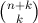# Invited speakers

The conference features three invited talks by Henry Cohn, Hendrik Lenstra, and Mohab Safey El Din and an invited software talk by Stephen Wolfram.

Henry Cohn, Microsoft Research New England, USA

### Solving equations with size constraints for the solutions

Abstract: In this talk, I'll survey some key applications within coding theory and cryptography for solving polynomial equations with size constraints on the solutions. Specifically, we seek solutions that are small integers or low-degree polynomials. In the single-variable case this is relatively well understood, thanks to important work in the late 1990's by Guruswami and Sudan and by Coppersmith, but higher dimensions hold many mysteries. I'll highlight connections with hot topics such as fully homomorphic encryption, as well as some problems for which progress should be possible.

Hendrik Lenstra, Universiteit Leiden, The Netherlands

### Lattices with symmetry

Abstract: It is a notoriously difficult algorithmic problem to decide whether a given lattice admits an orthonormal basis. However, this problem becomes doable if the lattice is given along with a suitably large abelian group of symmetries. The lecture is devoted to a precise formulation of this result and to an outline of the algorithm that underlies its proof. One of the ingredients is an elegant algorithmic technique that C. Gentry and M. Szydlo introduced several years ago in the context of cryptography, but that can be recast in algebraic language. Other ingredients are taken from analytic number theory and from commutative algebra. Part of the work reported on was done jointly with Alice Silverberg and René Schoof.

Mohab Safey El Din, Université Pierre et Marie Curie, Paris, France

### Critical Point Methods and Effective Real Algebraic Geometry: New Results and Trends

Abstract: Critical point methods are at the core of the interplay between polynomial optimization and polynomial system solving over the reals. These methods are used in algorithms for solving various problems such as deciding the existence of real solutions of polynomial systems, performing one-block real quantifier elimination, computing the real dimension of the solution set, etc.

The input consists of s polynomials in n variables of degree at most D. Usually, the complexity of the algorithms is (sD)O(nα) where α is a constant. In the past decade, tremendous efforts have been deployed to improve the exponents in the complexity bounds. This led to efficient implementations and new geometric procedures for solving polynomial systems over the reals that exploit properties of critical points. In this talk, we present an overview of these techniques and their impact on practical algorithms. Also, we show how we can tune them to exploit algebraic and geometric structures in two fundamental problems.

The first one is real root finding of determinants of n-variate linear matrices of size k × k. We introduce an algorithm whose complexity is polynomial in(joint work with S. Naldi and D. Henrion). This improves the previously known kO(n) bound. The second one is about computing the real dimension of a semi-algebraic set. It is a challenge to get an algorithm with complexity (sD)O(n), improving the long-standing (sD)O(n2) bound obtained by Koiran. I will present work in progress with E. Tsigaridas.

Stephen Wolfram, Wolfram Research Inc., USA.

### Computer Algebra: A 32-Year Update

Abstract: I last spoke at a computer algebra conference in August 1981. Since that time I created Mathematica (launched 1988) and Wolfram|Alpha (launched 2009). This talk will survey perspectives on computer algebra gained through these activities, as well as through my work in basic science. I will also describe what I see as being key future directions and aspirations for computer algebra.